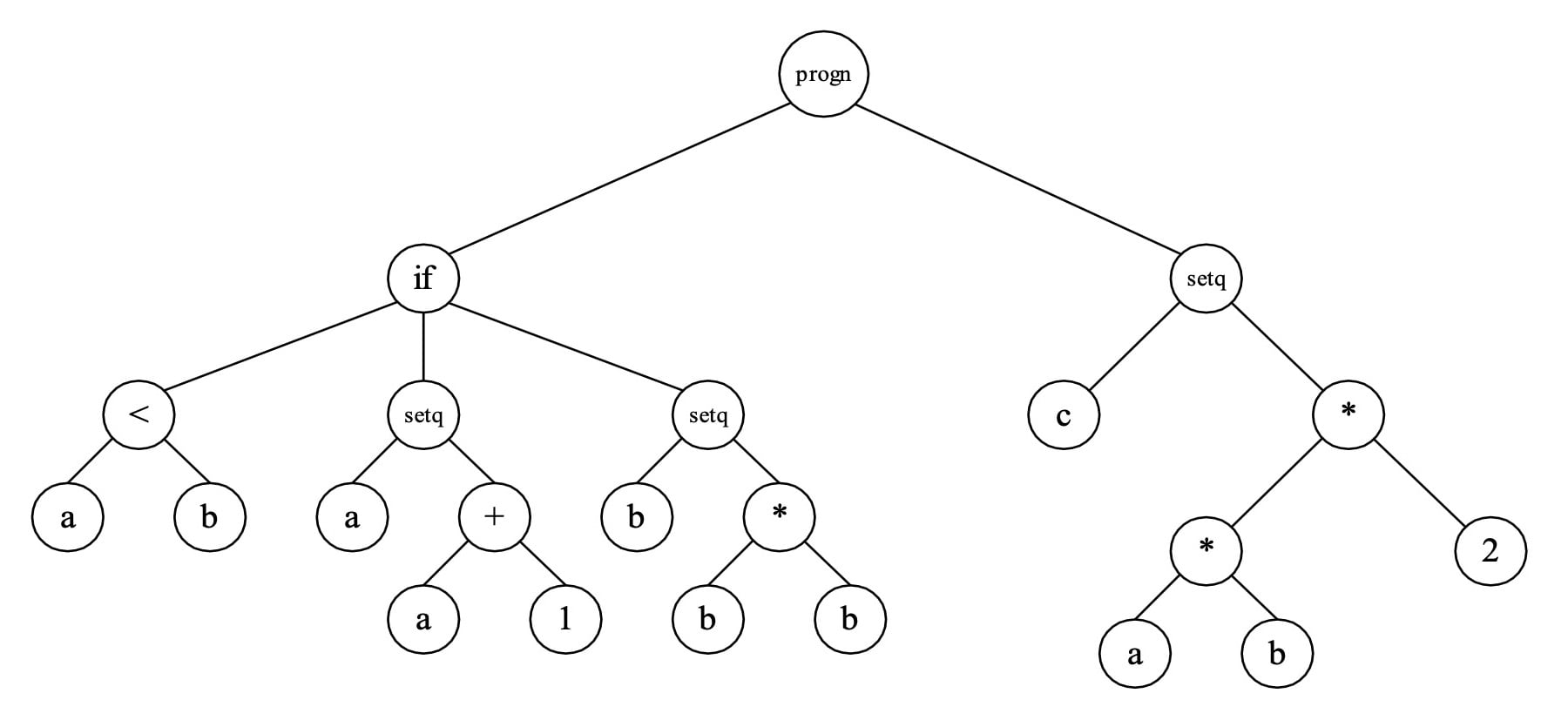Technical Article

# An Introduction to Genetic Programming: The “Holy Grail” of Systems that Can Author Themselves

December 19, 2019 by Greg Schmidt

## Can a system author itself? Genetic programming may represent the next revolution for control systems, robot navigation, pattern recognition, and more.

Perhaps the Holy Grail of computer science will have been discovered the day our machines are capable of writing their own programs. Genetic programming (GP) is a relatively new machine-learning paradigm that represents a step in that direction.

Genetic programming holds a great deal of promise in the realm of control engineering. In this article, we'll discuss what genetic programming is, how it can be represented, and take a look at an example program.

### Genetic Programming and Genetic Algorithms

GP is essentially a variation of the genetic algorithm (GA) originally conceived by John Holland. Like a GA, it is an evolutionary algorithm that relies on the application of genetic operators such as fitness proportionate reproduction, crossover, and mutation to drive a population of encoded programs or "individuals" through successive generations toward a solution.

However, unlike a GA which typically uses a fixed length bit string encoding, GP employs a variable-size, tree-based representation of an actual program. Therefore, the result of a successful GP run produces a computer program which, when given appropriate input and executed, yields the desired result.

Both Nichael Cramer and John Koza are credited for laying the foundations of GP. John Koza (who also holds a patent on GP) has done a significant amount of research on GP and his landmark book, "Genetic Programming" is considered the seminal work on the subject. Current research has demonstrated some encouraging successes of GP in a wide range of applications including robot navigation, game strategy acquisition, symbolic regression analysis, and control systems.

### Representation

The tree-based representation mentioned earlier is central to the GP theme as virtually any computer program may be represented in this way. In practice, a functional language such as LISP is well-suited to this form and it is easy to see how a LISP S-expression can be diagrammed as a tree (Figure 1).

Below, you’ll find three separate representations of the same information:

A simple program fragment:
BEGIN
IF a < b THEN a := a + 1 ELSE b := b * b
c := a * b * 2;

END

LISP equivalent:
(progn (if (a < b)
(setq a (+ a 1))
(setq b (* b b))
)
(setq c (* (* a b) 2))
)##### Figure 1. Tree representation of a program. Note that (progn arg1 arg2 arg3 ... argn) sequentially evaluates each argument.

The interior nodes of the tree consist of functions whereas the leaf nodes consist of terminals. Functions must have an argument count (commonly referred to as arity) of at least one, letting them be connected to other functions or terminals, thus providing the "glue" for branches within the tree.

Terminals typically include such things as constants and variables. Since terminals form the leaves of the tree, they always have an arity of zero. You are required to choose the set of functions and terminals for the problem you are attempting to solve. For example, the logical functions AND, OR, and NOT and the terminals X1 and X2, representing two boolean input variables, are appropriate if you are attempting to discover a program capable of synthesizing the XOR Boolean function. A fitness function is also required, since you must provide the means for one program to be measured against another in the sense that one is better at solving a given problem.

For example, in the XOR case, we may test the fitness of a program by executing the program once for each fitness case corresponding to the four possible Boolean inputs for X1 and X2 (0 0, 0 1, 1 0, 1 1) and adding up the number of correct responses (0, 1, 1, 0) respectively, for each test case.

Obviously, a program yielding a perfect score of four is considered a solution to the XOR problem, as shown in Listing 1.

A perfect solution to the XOR problem discovered by GP:
(defun program ()
(AND (OR X1 X2)
(NOT (AND X1 X2) )
)
)



### Next: Genetic Operators

In the next article, we'll take a look at the genetic operators that make evolutionary algorithms possible. We'll also use them in a more complex example algorithm.